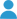By Rusty

# Calculator

This calculator determines the water depth to reach a given pressure or the pressure at a given depth. You can enter the depth or pressure input with most common units and it will be converted automatically. This calculator does not add air pressure at the surface – the results are relative to the surface pressure.

(success) Enter depth or pressure followed by unit. (e.g. "110 psi" or "450 ft")

Depth
Pressure

# Hydrostatic Water Pressure Formula

This calculator uses simple hydrostatic pressure equations. The pressure, $$P$$ of a fluid at depth depends only on the density, $$\rho$$, the acceleration of gravity, $$g$$, and the depth or height of the fluid column, $$h$$.

$P = \rho g h$

The calculator uses the following values for these parameters:

$$\rho_{fresh} = 997.0474 \: kg/m^3$$ (for freshwater)
$$\rho_{salt} = 1023.6 \: kg/m^3$$ (for saltwater)
$$g = 9.80665 \: m/s^2$$

For watertight enclosure design, please check out Under Pressure.

This calculator uses the js-quantities library by gentooboontoo for unit conversions.

## Authors### Rusty

Rusty is the founder and CEO of Blue Robotics. His background is in engineering but he likes to be involved in everything at Blue Robotics!